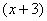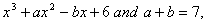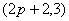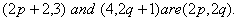# MATHEMATICS — 2004 (Set II — Compartment Delhi)

Except for the following questions, all the remaining questions have been asked in Set I.

Q. 4. Ifis a factor offind the values of a and b.

Q. 7. A loan has to be paid back in two equal annual instalments. If the rate of interest is 12% per annum, compounded annually, and each instalment is Rs. 3,920, find the loan and total interest charged.

Q. 8. A sewing machine is available for Rs. 7,000 cash or Rs. 2,000 as cash down payment followed by 4 monthly instalments of Rs. 1,300 each. Find the rate of interest under the instalment plan.

Q. 11. A two-digit number is seven times the sum of its digits and is also equal to 12 less than three times the product of its digits. Find the number.

Q. 16. The coordinates of the mid-point of the line joining the pointsandFind the values of p and q.

Q. 17. A card is drawn at random from a pack of 52 playing cards. Find the probability that the card drawn is neither a queen nor a jack.

Q. 24. The annual income of Kavita is Rs. 3,32,000 (exclusive of HRA). She contributes Rs. 5,000 per month towards her provident fund and pays an annual premium of Rs. 15,000 for her LIC policy. If she pays Rs. 4,000 per month towards income tax for the first eleven months of the year, find her income tax liability for the last month of the year.

for calculating income tax, use the following:

 (a) Standard Deduction Gross income Deduction (i) Upto Rs.75,000 (ii) From Rs.75,001 to Rs.5,00,000 (iii) More than Rs,5,00,000 40% of gross income Rs.30,000 Rs.20,000 (b) Rates of Taxes Slab Income tax (i) Upto Rs. 50,000 (ii) From Rs. 50,001 to Rs. 60,000 (iii) From Rs. 60,001 to Rs. 1,50,000 (iv) Rs. 1.50,000 and above No tax 10% of the amount exceeding Rs.50,000 Rs.1,000+20% of the amount Exceeding Rs.60,000 Rs.19,000+30% of the amount Exceeding Rs.1,50,000 (c) Rebate in Tax (i) 20% of the savings subject to a maximum of Rs. 14,000 if the gross income is upto Rs. 1,50,000. (ii)15% of the savings subject to a maximum of Rs. 10,500 if the gross income is from Rs. 1,50,001 to Rs. 5,00,000. (d) Rebate or women Assessers Rs.5000 over and other rebated
Maths 2004 Question Papers Class X
CBSE 2004 Question Papers Class X